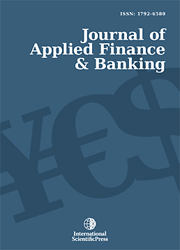# Journal of Applied Finance & Banking

#### Minimization of Value at Risk of Financial Assets Portfolio using Genetic Algorithms and Neural Networks

•[ Download ]
• Times downloaded: 1664
• Abstract

In this paper we have proposed an approach for minimization of a shares portfolio invested in a market which the fluctuations follow a normal distribution based in amathematical explicit formulae for calculating Value at Risk (VaR) for portfolios of linear financial assets invested using the Black-Scholes stochastic process and assuming that the portfolio structure remains constant over the considered time horizon. We minimize this Value at Risk using neural networks and genetic algorithms.ISSN: 1792-6599 (Online)
1792-6580 (Print)